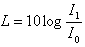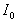Decibels

The intensity of sound is measured in a unit called the decibel (dB), which describes the relative intensity of a sound based on a logarithmic scale containing values ranging from 0 to 194.

A zero value on the decibel scale represents the weakest sound audible to humans and sound intensity increases in correspondence with numeric values. However, the relationship among the values on the decibel scale is not linear but logarithmic. A sound with a 50 dB level is not twice as intense as a sound with a 25 dB level. Rather, each three decibel increment affects a 50% change in sound pressure levels. Though reducing the decibel level produced by a sound source from 80 to 77 may not seem like a major change, it would actually represent a 50% reduction in audible sound.

The intensity of a sound reaching a person's ear depends not only on the intensity of the sound produced, but also on the persons distance from the source of the sound. If you were standing one foot away from a loud machine, for instance, you would experience higher decibel levels than if you were ten feet away, even though the intensity of the sound produced remains unchanged.

To calculate decibels, use the following formula:, where L is loudness andis 10-12 which is barely audible.